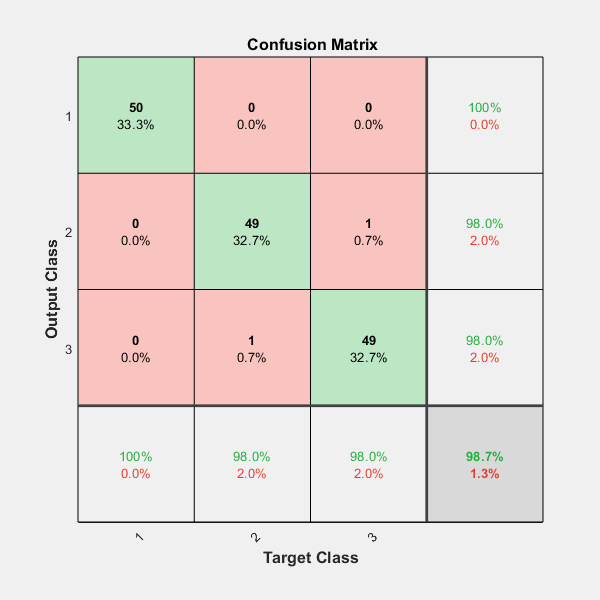# trainSoftmaxLayer

Train a softmax layer for classification

## Syntax

``net = trainSoftmaxLayer(X,T)``
``net = trainSoftmaxLayer(X,T,Name,Value)``

## Description

example

````net = trainSoftmaxLayer(X,T)` trains a softmax layer, `net`, on the input data `X` and the targets `T`.```
````net = trainSoftmaxLayer(X,T,Name,Value)` trains a softmax layer, `net`, with additional options specified by one or more of the `Name,Value` pair arguments.For example, you can specify the loss function.```

## Examples

collapse all

`[X,T] = iris_dataset;`

`X` is a 4x150 matrix of four attributes of iris flowers: Sepal length, sepal width, petal length, petal width.

`T` is a 3x150 matrix of associated class vectors defining which of the three classes each input is assigned to. Each row corresponds to a dummy variable representing one of the iris species (classes). In each column, a 1 in one of the three rows represents the class that particular sample (observation or example) belongs to. There is a zero in the rows for the other classes that the observation does not belong to.

Train a softmax layer using the sample data.

`net = trainSoftmaxLayer(X,T);`

Classify the observations into one of the three classes using the trained softmax layer.

`Y = net(X);`

Plot the confusion matrix using the targets and the classifications obtained from the softmax layer.

`plotconfusion(T,Y);`## Input Arguments

collapse all

Training data, specified as an m-by-n matrix, where m is the number of variables in training data, and n is the number of observations (examples). Hence, each column of `X` represents a sample.

Data Types: `single` | `double`

Target data, specified as a k-by-n matrix, where k is the number of classes, and n is the number of observations. Each row is a dummy variable representing a particular class. In other words, each column represents a sample, and all entries of a column are zero except for a single one in a row. This single entry indicates the class for that sample.

Data Types: `single` | `double`

### Name-Value Arguments

Specify optional pairs of arguments as `Name1=Value1,...,NameN=ValueN`, where `Name` is the argument name and `Value` is the corresponding value. Name-value arguments must appear after other arguments, but the order of the pairs does not matter.

Before R2021a, use commas to separate each name and value, and enclose `Name` in quotes.

Example: `'MaxEpochs',400,'ShowProgressWindow',false` specifies the maximum number of iterations as 400 and hides the training window.

Maximum number of training iterations, specified as the comma-separated pair consisting of `'MaxEpochs'` and a positive integer value.

Example: `'MaxEpochs',500`

Data Types: `single` | `double`

Loss function for the softmax layer, specified as the comma-separated pair consisting of `'LossFunction'` and either `'crossentropy'` or `'mse'`.

`mse` stands for mean squared error function, which is given by:

`$E=\frac{1}{n}\sum _{j=1}^{n}\sum _{i=1}^{k}{\left({t}_{ij}-{y}_{ij}\right)}^{2},$`

where n is the number of training examples, and k is the number of classes. ${t}_{ij}$ is the ijth entry of the target matrix, `T`, and ${y}_{ij}$ is the ith output from the autoencoder when the input vector is xj.

The cross entropy function is given by:

`$E=\frac{1}{n}\sum _{j=1}^{n}\sum _{i=1}^{k}{t}_{ij}\mathrm{ln}{y}_{ij}+\left(1-{t}_{ij}\right)\mathrm{ln}\left(1-{y}_{ij}\right).$`

Example: `'LossFunction','mse'`

Indicator to display the training window during training, specified as the comma-separated pair consisting of `'ShowProgressWindow'` and either `true` or `false`.

Example: `'ShowProgressWindow',false`

Data Types: `logical`

Training algorithm used to train the softmax layer, specified as the comma-separated pair consisting of `'TrainingAlgorithm'` and `'trainscg'`, which stands for scaled conjugate gradient.

Example: `'TrainingAlgorithm','trainscg'`

## Output Arguments

collapse all

Softmax layer for classification, returned as a `network` object. The softmax layer, `net`, is the same size as the target `T`.

## Version History

Introduced in R2015b